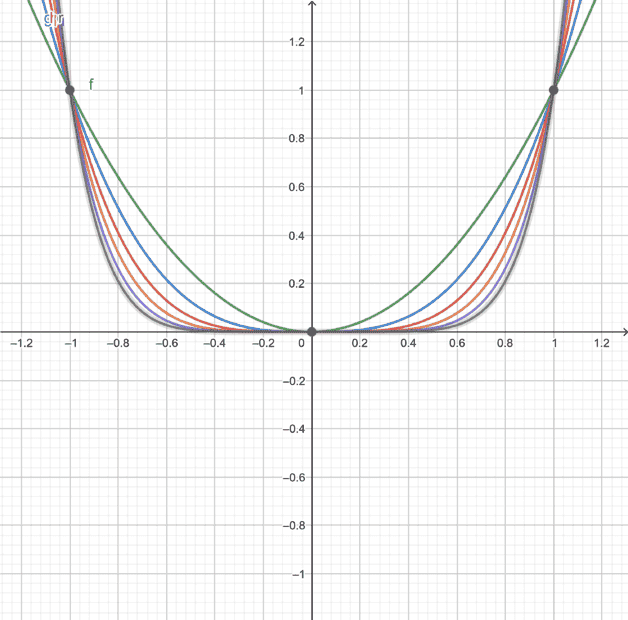# Pattern of variables with absolute value exponents

• mcastillo356

#### mcastillo356

Gold Member
Homework Statement
Want to prove that, raised to an absolute value, monomials behave bounded
Relevant Equations
Variables raised to natural numbers in absolute value (for my purpose, I do not consider 0 as belonging to naturals)
On ##x\in{(-1,1)}##, ##x\in{\mathbb{R}}##, ##\forall{n\in{\mathbb{N}}}##, ##x^{|2n|}=O(x^{|2n+1|})##Greetings, PF

I don't think the question is well defined as written.

Are you looking for something like ##x^n## is bounded in (-1,1)?

•mcastillo356
Since n is a natural number (##n \in \mathbb N##), what is the purpose of absolute values on the exponents? The natural numbers are integers (no fractional part) that are greater than zero, although one definition also includes zero.
With this in mind, ##x^{|2n|} = x^{2n}##, and similarly for ##x^{|2n + 1|}##.

•mcastillo356
I don't think the question is well defined as written.

Are you looking for something like ##x^n## is bounded in (-1,1)?
Yes!
(i) ##x^2\leq{x^{2+n}}##, ##\forall{n\in{\mathbb{N}}}##
(ii) We write ##f(x)=O(u(x))## as ##x\rightarrow{a}## provided that $$|f(x)|\leq{k|u(x)|}$$ holds for some constant ##k## on some open interval containing ##x=a##
(iii) ##|x^2|\leq{k|x^{2+n}|}##, for ##k=1##
(iv) ##x^2=O(x^{2+n})####x^2\leq{x^{2+n}}## ?? On ##x\in{(-1,1)}## ??
Am I misssing something essential ?

##\ ##

##x^2\leq{x^{2+n}}## ?? On ##x\in{(-1,1)}## ??
Am I misssing something essential ?

##\ ##
Thanks indeed

@BvU, it is ##x^{2+n}\leq{x^2}## on ##x\in{(-1,1)}##, ##\forall{n\in{\mathbb{N}}}##

Consequently, #4 must be quoted again, arranged:
(i) ##x^2\leq{x^{2+n}}##, ##\forall{n\in{\mathbb{N}}}##
(ii) We write ##f(x)=O(u(x))## as ##x\rightarrow{a}## provided that $$|f(x)|\leq{k|u(x)|}$$ holds for some constant ##k## on some open interval containing ##x=a##, (##x=0## in this case)
(iii) ##|x^{2+n}|\leq{k|x^2|}##, for some constant ##k##
(iv) ##x^{2+n}=O(x^2)##, e.g. there is a bound: ##x^2##, ##\forall{n\in{\mathbb{N}}},\; x\in{(-1,1)},\; x\rightarrow{0}##
Right? Greetings, PF. Please, check the LaTeX

Last edited: